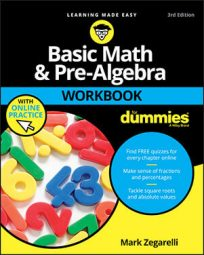##### Basic Math & Pre-Algebra Workbook For Dummies with Online PracticeTo successfully study pre-algebra, understand that a specific order of operations needs to be applied. Also recognize some basic math principles, such as the ability to recognize and understand mathematical inequalities, place value, absolute value, and negation.

## Mathematical order of operations

The rules for deciding the order to evaluate arithmetic expressions, no matter how complex, are called the order of operations. The complete order of operations in math is:

1. Contents of parentheses (and other grouping symbols) from the inside out

2. Powers from left to right

3. Multiplication and division from left to right

4. Addition and subtraction from left to right

## Mathematical inequalities

When two numbers are different in value, a variety of symbols are used to turn them into an inequality. The four most common inequalities in arithmetic are:

• Greater than (>) means that the first number or expression is greater than the second:

• 5 > 4

• 1,000 > 100

• 2 > –2

• Less than (<) means that the first number or expression is less than the second:

• 7 < 9

• 1,776 < 1,777

• –5 < 0

• Doesn’t equal (≠) means that the two numbers or expressions aren’t equal:

• 2 ≠ 3

• 3 ≠ 2

• –17 ≠ 17

• Approximately equals (≈) means that the two numbers or expressions are close in value:

• 999 ≈ 1,000

• 14.001 ≈ 14

• 1,000,823 ≈ 1,000,000

## Place value

Our number system (Hindu-Arabic) has ten familiar digits, 0 to 9. Numbers higher than 9 are made using place value, which assigns a digit a greater or lesser value depending on where it shows up in a number. For example, 3,000 + 600 + 10 + 9 + 0.8 + 0.04 + 0.002 = 3,619.842.

Thousands Hundreds Tens Ones Decimal Point Tenths Hundredths Thousandths
3 6 1 9 . 8 4 2

## Understanding absolute value and negation

The positive value of a number is its absolute value. It tells you the distance of a number from zero on the number line. Placing bars (| |) around a number or expression gives you its absolute value:

• The absolute value of a positive number is positive. The absolute value of 8 is |8|, which equals 8.

• The absolute value of a negative number is positive. The absolute value of –8 is |–8|, which equals 8.

Attaching a minus sign (–) negates a number or expression:

• Negating a positive number makes it negative. To negate the number 3, attach a minus sign to it, changing it to –3.

• Negating a negative number makes it positive. To negate the number –3, attach a minus sign to it, changing it to –(–3), which equals 3.

## Basic algebra conventions

In algebra, a letter such as x that takes the place of a number in one or more equations is called a variable. Practices you need to know about how algebra problems are written:

• To add up many of the same variable, count the number of times that variable appears, and then attach that number (called a coefficient) to that variable:

• x + x + x + x + x = 5x

• To multiply many of the same variable, count how many times the variable appears, and then attach an exponent to that variable:

• x · x · x · x = x4

• Write division problems as fractions:

• x ÷ 2 is written as x/2

## Solving an algebraic equation

An algebraic equation is an equation with at least one variable. Apply operations equally to both sides to modify an equation. For example, you can add 2 to both sides, subtract x from both sides or multiply both sides by 2. To solve an algebraic equation, apply operations that isolate x on one side of the equation and put a number on the other side. Here are the standard steps for a problem such as 10x – 6 = 7x + 9:

1. Add or subtract to move all the constants to one side of the equation.

If you add 6 to both sides, the –6 cancels out on the left: 10x = 7x + 15.

2. Add or subtract to move all the variables to the other side.

If you subtract 7x from both sides, the 7x cancels out on the right: 3x = 15.

3. Divide both sides by the coefficient (the number next to the variable) to isolate x.

Dividing both sides by 3 gives you the answer x = 5.